# Mathematics Simplified

By Anjali Sharma
Maths is often dreaded as a subject by most of the students. Here is an attempt to simplify various topics in Mathematics and help reduce Maths Phobia.
##### Where to listen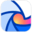Breaker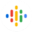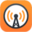Overcast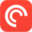Pocket Casts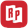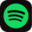Spotify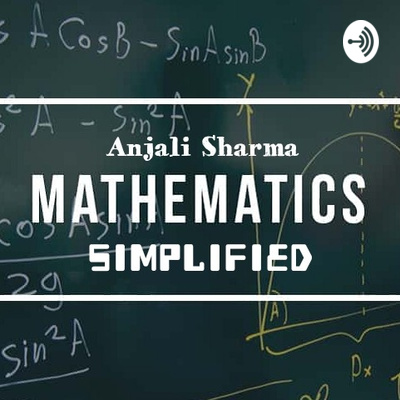INTRODUCTION TO PROBABILITY
Probability theory is an important and integral part of study of Mathematics. It is necessary for the learners to understand the basic terminology before commencing with the rigorous exercises
03:54
October 16, 2021The Exam Plan
Getting a few tips to achieve success in the exam is what every student strives for. Here are some simple yet effective tips to ace the examination time
04:02
October 2, 2021GEOMETRIC PROGRESSIONS
Geometric Progression is a special type of sequence which is important in the study of sequence and series.
06:07
September 6, 2021MATHEMATICAL MODELLING-3
The real life use of Mathematical Modelling is seen in our lives.In present time,data analysis and Mathematical Modelling are helping scientists and researchers to get correct cures for Corona
04:57
July 21, 2021MATHEMATICAL MODELLING - 2
Continuing with the concept of Mathematical Modelling, let's hope that this will make you understand what steps are involved in making a Mathematical model
03:56
June 10, 2021Mathematical Modelling - 1
Mathematical Modelling is a process to convert real life problems to mathematical ones and find solutions mathematically.
03:49
May 30, 2021EQUIVALENCE RELATIONS
Mathematical relations are simple to understand if we use examples to make them relatable. Let's hope that this endeavour helps listeners in understanding Equivalence Relations
04:34
March 28, 202104:30
February 8, 2021Arithmetic Progressions
Arithmetic Progressions or AP are special sequences which are characterised by the special formulae for the general term. We can identify whether a given sequence is an AP or not by using the formulae.
04:30
December 6, 2020INTRODUCTION TO SEQUENCES
Sequences are an integral and interesting part of the mathematical world. While some follow specific mathematical rules, others may be just an array of elements. Let's hope that this introduction will help you to get an insight into the Sequence world.
04:23
November 20, 2020Relations
Mathematical relations are an important part of the Set Theory. Also known as the Subsets of the Cartesian product of sets, we have a variety of Relations which are defined on different sets.
04:19
September 21, 2020Cartesian Product of Sets
Given two sets, we can form the Cartesian product as the set of all the ordered pairs. As the name Cartesian Plane is familiar, we will be able to understand the concept.
03:49
August 19, 2020Subsets
Study of Subsets is an integral part of the Set Theory. Let's hope we can understand the concept in a better way.
03:00
May 17, 2020Types of Sets
There are different ways of writing the set. This is a small piece describing them and the various types of Sets.
06:34
May 10, 2020Sets Introduction
Set theory is an important and integral part of Mathematics. This episode gives you a basic idea of the definition of Sets.
03:39
May 9, 2020